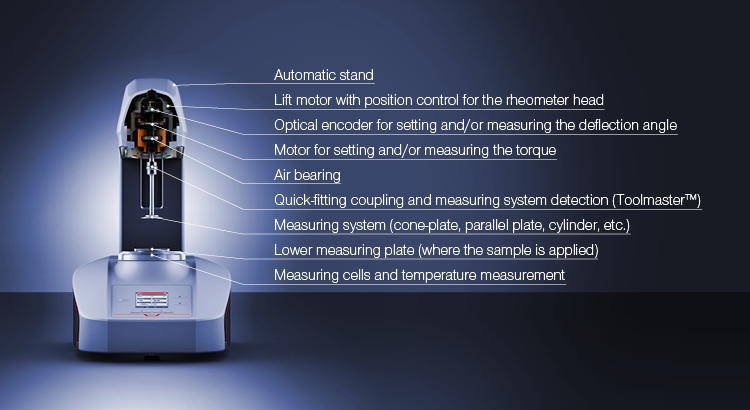4 Rates

# Rheological measurements

Every substance has rheological properties. These properties can be determined with a rheometer.

Rheology and its effects are visible everywhere: Whenever a material flows or is being deformed, rheology is involved. Rheology is the science of the flow and deformation behavior of substances. When matter flows or is deformed there are three factors which must be considered: the internal structure, the external forces which act on the substance, and the environmental conditions, for example temperature.

## The rheometer

To measure the rheological properties of a material, rheometers are used. They measure the torque and the deflection angle of the measuring bob. This means that in a viscosity measurement the rheometer presets a certain current that correlates to a defined torque. The sample provides resistance or a reset torque to the setting and the resulting deflection angle is then measured very precisely by the rheometer’s encoder. The speed is calculated from the deflection angle and the time. In a measurement, modern rheometers can set either the torque or the speed – the other parameter is then measured. In fact, it is always the current – and therefore the torque – which is provided and the parameter of either speed or torque is set via a quick controller. The speed is then measured. However, the rheometer user is usually more interested in the rheological parameters. Rheological parameters are calculated from the measured values torque, deflection angle, and speed using conversion factors (see Table 1). All further parameters, such as viscosity, are not measured but calculated.

Measurement valueConversion factorRheological parameters
Torque M [mNm]CSS-factorShear stress τ [Pa]

CSD-factor

Deformation γ [%]
Speed n [min-1]CSR-factorShear rate  $\dot{\gamma}$ [s-1]

Table 1: Measuring values and rheological parameters with the conversion factors

### The rheometer setup

The measuring head of a rheometer contains the drive motor and encoder which measure and set the previously mentioned torque, deflection angle, and speed.Figure 1: Setup of a modern air-bearing rotational and oscillatory rheometer with the most important components

Basically there are two measuring methods available: Rotational tests and oscillatory tests. With rotational measurements the measuring bob turns in one direction. In an oscillatory test the measuring bob “oscillates” around the axis.

When measuring ketchup for instance, its viscosity should be measured with a shear-rate-controlled rotational test. The user sets the shear rate profile – the different speeds of rotation – and the rheometer determines the required shear stress. The viscosity η is then calculated in the rheometer software according to the viscosity law from the quotients of the shear stress τ and shear rate $\dot{\gamma}$..

As mentioned, the shear stress correlates with the torque. If the ketchup is measured with a large measuring system, a large torque is required because the shear surface is large. If a small measuring system is used, a smaller torque is needed to obtain the required speed. As the rheological parameter shear stress τ is calculated from the torque in relation to the shearing surface, the shear stress is therefore independent of the used measuring system. It is therefore easier to work with the rheological parameters. The same is true for the shear rate, which correlates with the speed. If in this case ketchup is measured in two different measuring systems, one with a large shear gap and one with a narrow shear gap, the same rotational speed results in a lower shear rate in the system with the large gap than in the system with the narrow gap. As with the calculation of the shear stress, for the calculation of the rheological parameter ‘shear rate’ the influence of the measuring system is considered using a conversion factor:

Shear rate = speed / gap distance

The viscosity values are calculated from the rheological parameters which are independent of the instrument:

Viscosity = shear stress / shear rate

If jello was in the shear gap instead of ketchup, the aim of the test would be, for example, to investigate the viscoelastic behavior and the characterization of the undestroyed structure at rest. In this case a rotational test would not be very useful. Instead, oscillatory tests with set sinusoidal oscillation of the measuring system and the sample are carried out. Also here, the rheometer determines the deflection of the measuring bob and the required torque. However, because jello has viscoelastic properties the response wave is time-delayed compared to the set oscillation. This time delay is called the phase shift δ.

The values measured by the rheometer (deflection angle, torque, and phase shift) together with the conversion factors for the measuring system now give all necessary data to calculate the required rheological parameters such as the storage modulus G’ or loss modulus G’’.

## Further parameters measured with rheometers

The temperature, which is an extremely important parameter, is measured directly in the measuring cell very close to the sample. Exact temperature control is very important because most rheological parameters are greatly affected by the temperature. The viscosity of a calibrating oil, for example, changes by approx. 7 % when there is a temperature increase of just 1 °C.